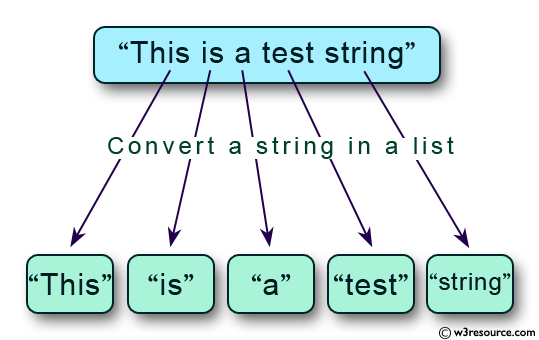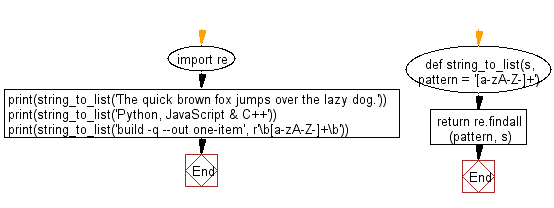﻿ Python: Convert a given string into a list of words - w3resource# Python: Convert a given string into a list of words

## Python String: Exercise-46 with Solution

Write a Python program to convert a given string into a list of words.Sample Solution-1:

Python Code:

``````str1 = "The quick brown fox jumps over the lazy dog."
print(str1.split(' '))
str1 = "The-quick-brown-fox-jumps-over-the-lazy-dog."
print(str1.split('-'))
```
```

Sample Output:

```['The', 'quick', 'brown', 'fox', 'jumps', 'over', 'the', 'lazy', 'dog.']
['The', 'quick', 'brown', 'fox', 'jumps', 'over', 'the', 'lazy', 'dog.']
```

## Visualize Python code execution:

The following tool visualize what the computer is doing step-by-step as it executes the said program:

Sample Solution-2:

• Use re.findall() with the supplied pattern to find all matching substrings.
• Omit the second argument to use the default regexp, which matches alphanumeric and hyphens.

Python Code:

``````import re
def string_to_list(s, pattern = '[a-zA-Z-]+'):
return re.findall(pattern, s)
print(string_to_list('The quick brown fox jumps over the lazy dog.'))
print(string_to_list('Python, JavaScript & C++'))
print(string_to_list('build -q --out one-item', r'\b[a-zA-Z-]+\b'))
```
```

Sample Output:

```['The', 'quick', 'brown', 'fox', 'jumps', 'over', 'the', 'lazy', 'dog']
['Python', 'JavaScript', 'C']
['build', 'q', 'out', 'one-item']
```

Flowchart:## Visualize Python code execution:

The following tool visualize what the computer is doing step-by-step as it executes the said program:

Python Code Editor:

Have another way to solve this solution? Contribute your code (and comments) through Disqus.

What is the difficulty level of this exercise?

Test your Programming skills with w3resource's quiz.

﻿

## Python: Tips of the Day

Check if a given key already exists in a dictionary:

In is the intended way to test for the existence of a key in a dict.

```d = {"key1": 10, "key2": 23}

if "key1" in d:
print("this will execute")

if "nonexistent key" in d:
print("this will not")
```

If you wanted a default, you can always use dict.get():

```d = dict()

for i in range(100):
key = i % 10
d[key] = d.get(key, 0) + 1
```

and if you wanted to always ensure a default value for any key you can either use dict.setdefault() repeatedly or defaultdict from the collections module, like so:

```from collections import defaultdict

d = defaultdict(int)

for i in range(100):
d[i % 10] += 1
```

but in general, the in keyword is the best way to do it.

Ref: https://bit.ly/2XPMRyz